Chapter 4: Inequalities

# Graphing a 2D Inequality

To graph an inequality, borrow the strategy used to draw a line graph in 2D. To do this, replace the inequality with an equal sign.

Example 4.4.1

Consider the following inequalities:

$\begin{array}{rrrrr}2x&+&2y&<&12 \\ 2x&+&2y&\le&12 \\2x&+&2y&>&12 \\2x&+&2y&\ge&12 \end{array}$

All can be changed to $3x + 2y = 12$ by replacing the inequality sign with =.

It is then possible to create a data table using the new equation.

Create a data table of values for the equation $3x + 2y = 12.$

$x$ $y$
0 6
2 3
4 0
6 −3

Using these values, plot the data points on a graph.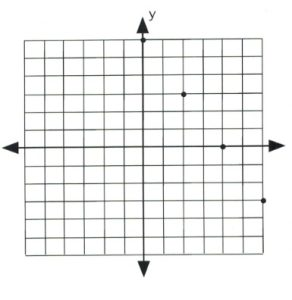Once the data points are plotted, draw a line that connects them all. The type of line drawn depends on the original inequality that was replaced.

If the inequality had ≤ or ≥, then draw a solid line to represent data points that are on the line.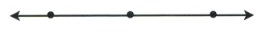If the inequality had < or >, then draw a dashed line instead to indicate that some data points are excluded.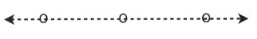If the inequality is either $3x + 2y \le 12$ or $3x + 2y \ge 12$, then draw its graph using a solid line and solid dots.If the inequality is either $3x + 2y < 12$ or $3x + 2y$ > $12$, then draw its graph using a dashed line and hollow dots.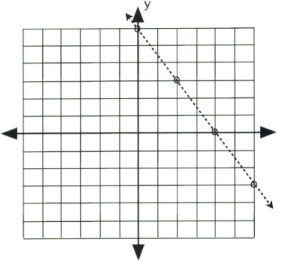There remains only one step to complete this graph: finding which side of the line makes the inequality true and shading it. The easiest way to do this is to choose the data point $(0, 0)$.

It is evident that, for $3(0) + 2(0) \le 12$ and $3(0) + 2(0) < 12$, the data point $(0, 0)$ is true for the inequality. In this case, shade the side of the line that contains the data point $(0, 0)$.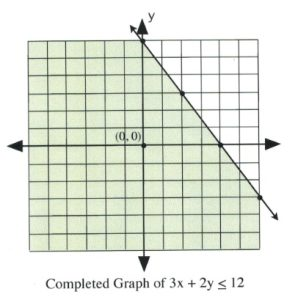It is also clear that, for $3(0) + 2(0) \ge 12$ and $3(0) + 2(0)$ > $12$, the data point $(0, 0)$ is false for the inequality. In this case, shade the side of the line that does not contain the data point $(0, 0)$.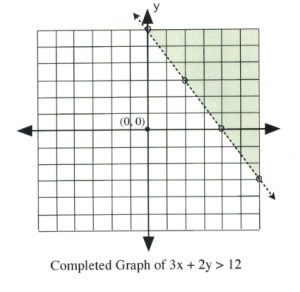# Graphing an Absolute Value Function

To graph an absolute value function, first create a data table using the absolute value part of the equation.

The data point that is started with is the one that makes the absolute value equal to 0 (this is the $x$-value of the vertex). Calculating the value of this point is quite simple.

For example, for $| x - 3 |$, the value $x = 3$ makes the absolute value equal to 0.

Examples of others are:

$\begin{array}{rrrrrrrrr} |x&+&2|&=&0&\text{when}&x&=&-2 \\ |x&-&11|&=&0&\text{when}&x&=&11 \\ |x&+&9|&=&0&\text{when}&x&=&-9 \\ \end{array}$

The graph of an absolute value equation will be a V-shape that opens upward for any positive absolute function and opens downward for any negative absolute value function.

Example 4.4.2

Plot the graph of $y = | x + 2 | - 3.$

The data point that gives the $x$-value of the vertex is $| x + 2 | = 0,$ in which $x = -2.$ This is the first value.

For $x = -2, y = | -2 + 2 | - 3,$ which yields $y = -3.$

Now pick $x$-values that are larger and less than −2 to get three data points on both sides of the vertex, $(-2, -3).$

$x$ $y$
1 0
0 −1
−1 −2
−2 −3
−3 −2
−4 −1
−5 0

Once there are three data points on either side of the vertex, plot and connect them in a line. The graph is complete.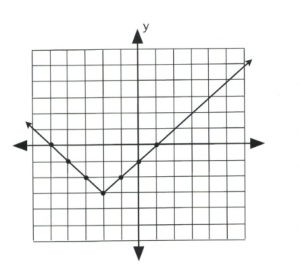Example 4.4.3

Plot the graph of $y = -| x - 2 | + 1.$

The data point that gives the $x$-value of the vertex is $| x - 2 | = 0,$ in which $x = 2.$ This is the first value.

For $x = 2, y = -| 2 - 2 | + 1,$ which yields $y = 1.$

Now pick $x$-values that are larger and less than 2 to get three data points on both sides of the vertex, $(2, 1).$

$x$ $y$
5 −2
4 −1
3 0
2 1
1 0
0 −1
−1 −2

Once there are three data points on either side of the vertex, plot and connect them in a line. The graph is complete.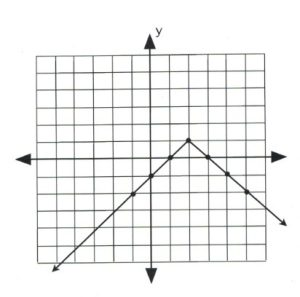# Questions

For questions 1 to 8, graph each linear inequality.

1. $y$ > $3x + 2$
2. $3x - 4y$ > $12$
3. $2y \ge 3x + 6$
4. $3x - 2y \ge 6$
5. $2y$ > $5x + 10$
6. $5x + 4y$ > $-20$
7. $4y \ge 5x + 20$
8. $5x + 2y \ge -10$

For questions 9 to 16, graph each absolute value equation.

1. $y=|x-4|$
2. $y=|x-3|+3$
3. $y=|x-2|$
4. $y=|x-2|+2$
5. $y=-|x-2|$
6. $y=-|x-2|+2$
7. $y=-|x+2|$
8. $y=-|x+2|+2$